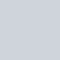46. Permutations

medium

# 個人範例程式碼

``````class Solution:
def permute(self, nums: List[int]) -> List[List[int]]:
if not nums:
return nums

self.ans = []
self.dfs(nums, [])
return self.ans

def dfs(self, nums, permutations):
# end of recursion
if not nums:
self.ans.append(list(permutations)) # deepcopy
return

# define and split
for i, num in enumerate(nums):
permutations.append(num)
# temporarily remove element i = start[0:i] + start[i+1:]
self.dfs(nums[:i] + nums[i+1:], permutations)
permutations.pop() # backtracking
``````

# Reference### 2 則留言

1. #### 【Leetcode】python -  Permutations II 個人解法筆記 #重要題型 - 嗡嗡的隨手筆記

[…] 【Leetcode】python –  Permutations 個人解法筆記 #重要題型 […]

2. #### Best 23 46. Permutations Python Honda Anh Dũng

[…] Quote from the source: … […]